## Tuesday, May 28, 2013

### The Data are In!

Note: In this post I'll be referencing a couple papers, citations for which can be found at the post's end.

It's been a long time and a couple of science fairs since my last post, so I thought I'd take the time to update you on what I've been up to these past few months. With the end of the school year (and my high school career!) consuming a lot of my time, I haven't gotten to work on the project as much as I would have liked; in fact, I'm still not done experimenting or collecting data. I'll summarize the data I've collected so far and the methods I've used to collect it below.A sample water arc explosion. Note the large fog jet and the bright arc inside the accelerator barrel.
Since I got my water explosion machine working reliably, I've been primarily concerned with quantifying the water explosions' energy inputs and outputs. The former has been relatively straightforward, as it only requires a couple of oscilloscope measurements (below). In each explosion I looked at, I measured the capacitor voltage before and after discharge, which I used to calculate the electrical energy delivered to the explosion chamber, as well as the peak discharge current, which is predicted to correlate with explosion strength (Graneau et al., 2000).Sample capacitor voltage waveform. Each horizontal division represents 5 μs and each vertical division represents 5 kV. The voltage before discharge is about 15 kV.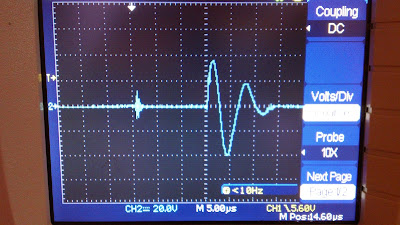Sample discharge current waveform. Each horizontal division represents 5 μs and each vertical division represents approximately 2.5 kA. The peak current is 4.5 kA.

I've made some minor tweaks to my charging circuit. I've also made this handy illustration to show them off (click for higher resolution):

The the EMF-sensitive shut-off circuit, which cuts power to the H-bridge inverter when it detects that the capacitors have discharged, now triggers more reliably and the switching transistors (in the H-bridge) are less prone to overheating.

The latest incarnation of my water explosion chamber is pictured below:

The chamber comprises a cylindrical cup with an open top and two electrodes. One electrode projects through the bottom of the cup and the other forms a ring around the inside of the chamber. It's made from a giant block of wood because all the plastics I had available weren't strong enough to make a durable explosion chamber out of.

To determine a water explosion’s kinetic energy, I employed the same projectile-based method used by physicist Peter Graneau in his research on water explosions (Graneau et al., 2000). The idea is to place a porous projectile (mine was made of balsa wood) over the muzzle of the accelerator barrel so that a water explosion within the barrel launches the projectile into the air and some of the fog penetrates into the projectile during the process. The height to which the projectile ascends can be measured and used to estimate the initial kinetic energy (in the vertical direction) of the explosion.

Now for the maths: Let the balsa wood projectile have mass M and let the explosion launch it to a height h. Let m0 be the total mass of the expelled water, m1 be the mass of the water that bounces off the projectile, and m2 be the mass of the water absorbed by the projectile. The collision of m1 with M is elastic, and the collision of m2 with M is inelastic. Say m1 has initial average velocity μ01 and m2 has initial average velocity μ02. After the collision of m1 with M, say m1 has average velocity μf1 and M has velocity v1. After the collision of m2 with M, say the combined mass M+m2 has velocity v2.

M, m0, and m2 can be determined by before and after measurements of the projectile mass and water mass. h can be measured by taking a video recording of the explosion and analyzing the video frame-by-frame. The other quantities of interest, namely m1, μ01, and μ02, must be found indirectly.

Energy and momentum conservation in the collision of m1 with M require that: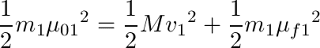Eqn. 1

and momentum conservation in the collision of m2 with M requires that:Eqn. 2
Examining the projectile, it follows from the conservation of mechanical energy that:Eqn. 3
where g is the acceleration due to gravity. Solving (3) for v2 yields:Eqn. 4
By making some assumptions about the fog jet’s mass and velocity distributions, we can use (1), (2), and (4) to solve for m1, μ01, and μ02 and then calculate the fog explosion’s kinetic energy.

We can obtain a variety of approximations for the explosion’s kinetic energy using different models for the explosion’s velocity distribution. Each of the below velocity-mass functions could plausibly describe a water arc explosion given the behavior of the fog jets in the high-speed images presented in Hathaway et al. (1998).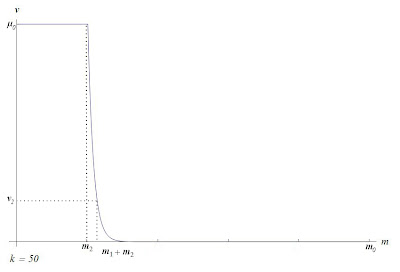Sample graphs of the velocity-mass function v(m) for a water explosion. The first has decay rate k = 5, while the second has k = 50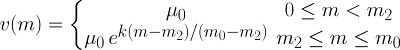Eqn. 5

Using the methods described above, I was able to compute the input and output energies of several water explosions. A sampling of the data I collected is presented in Table 1 below (click to enlarge).

Table 1: The input and output energy data of 8 shots using distilled water at room temperature. In all shots, the charging capacitance C was 0.6 μF. The capacitor input energy was computed as E0 =  C/2*(V0^2 - Vr^2).Key: V0: initial capacitor charging voltage; Vr: residual capacitor voltage after discharge; E0: electrical input energy; Ef: kinetic output energy; Ef/E0: the explosion’s efficiency, the ratio of output to input energy. M, m0, m2, and h are as defined in the Methods section. All kinetic energies are computed using a v(m) model with k = 300.

The results in Table 1 indicate that water arc explosions liberate stored potential energy (though these results are only as good as the assumptions made in obtaining them).  Looking at the last 3 columns of Table 1, it can be seen that the water explosion’s kinetic energy was even greater than the pulse discharge’s electrical energy in three of the eight shots displayed. These results are consistent with the findings reported in Graneau et al. (2000) and require that some sort of internal water energy is released during a water arc explosion.

If electrodynamic forces alone were responsible for the explosions, their kinetic energies should be only a fraction of the total input energy. For a typical shot with E= 60 J, a peak discharge current of 10 kA, and a pulse width of 10 μs,  the upward Lorentz pinch thrust should be about 0.1 N on average, corresponding to an explosion kinetic energy of 2.5 nJ = 2.5 x 10^(-9) J. This is about ten orders of magnitude off the observed values for Ein Table 1.

Note that the accuracy of a kinetic energy measurement depends largely on the explosion’s actual velocity-mass distribution. If this actual distribution differs significantly from the model function in eqn. (5), the calculated value for Ef may significantly over- or underestimate the explosion’s actual kinetic energy. Furthermore, the parameter k in the velocity model (Eqn. 5) will have a significant impact on the calculated kinetic energies. In table 1 k is taken to be 300; a lower value of k will yield lower Ef. A more sophisticated measurement system (e.g. a phase Doppler particle analyzer) for determining a fog explosion’s velocity and mass distributions would be needed to calculate Ef with greater accuracy and eliminate the uncertainty surrounding k.

So, if water explosions do in fact release energy, where does this energy come from? I can't yet give you a definitive answer, but I have a hunch that the energy of a water explosion comes from the reorganization of the water’s hydrogen bonding network during the explosion process. Inelastic neutron scattering experiments have revealed the existence of two different kinds of hydrogen bonds in water, each bond with a different strength (Li and Ross, 1993). The “strong” and “weak” bonds have vibrational force constants of 32 meV and 24 meV, respectively, and exist in water in a strong-to-weak ratio of about 2:1 (Graneau, 1998). During an explosion event, forces associated with the high-current arc discharge tear the water into fog droplets. This consumes a certain amount of mechanical energy, which is stored as additional surface tension energy in the fog. However, the molecules in the fog droplets now have significantly fewer neighbors than they did in the bulk water (see my cheesy little diagram below) and can more easily reorient themselves into lower energy states. Thus, weak hydrogen bonds can reform into strong hydrogen bonds, and van der Waals attractions can collapse into weak hydrogen bonds. This bond reformation would, of course, release energy, and could conceivably supply the kinetic energy of a water explosion.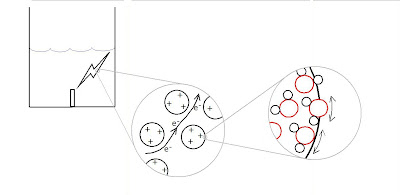For a water explosion to release potential bond energy, as described above, the post-explosion fog must be in a lower-energy state (i.e. contain stronger intermolecular bonds) than the pre-explosion water. When the fog reforms into bulk water, it must therefore absorb energy from its surroundings to restore itself to its original higher-energy state. In other words, the fog must absorb heat as it agglomerates back into bulk water (this is rather unusual, as condensation is generally an exothermic process).

In light of this, I set out to determine the amount of heat (if any) absorbed by a quantity of post-explosion fog as it agglomerates back into bulk water. To do this, I strapped a balsa wood absorber over the muzzle of the accelerator barrel. The absorber would capture most of the water expelled by an explosion in the barrel. Before an explosion event, I would measure the temperatures of the water and balsa wood absorber , and after an explosion, I would measure the final temperature of the water—balsa-wood system by inserting a temperature probe into the absorber. Knowing these temperatures and the heat capacities of the water, balsa wood, and temperature probe, I could calculate and compare the initial and final thermal energies of the water—balsa-wood—temperature-probe system , revealing whether any heat was absorbed by the water after the explosion. In these calculations, I tried to account for the resistive heating that takes place in the water arc during an explosion; I calculated this heat using the current discharge's pulse width and underdamped ringing frequency.

Table 2 below displays the thermal energy measurements for several water explosions (click to enlarge).

Table 2: The initial and final thermal energy data for 5 shots using distilled water at room temperature.  The charging capacitance was 0.6 μF for all shots.Key: mw: mass of the water involved in the heat exchange; Tw: initial water temperature; mb: mass of the balsa wood involved in the heat exchange; Tb: initial balsa wood temperature; Tf: final temperature of the water—balsa-wood—temperature-probe system (“the system”); QR: ohmic heat accrued by the water during the capacitor discharge; Qi: initial thermal energy of the system; Qf: final thermal energy of the system; ΔQ = Qi – Qf.

Looking at the last three columns, one can see that the post-explosion fog does indeed absorb energy. For each shot measured, the thermal energy of the water—balsa-wood—temperature-probe system decreased significantly over the course of the explosion event. The difference between the initial and final thermal energies was about 75 J on average, which is sufficiently large to account for the explosion kinetic energies reported in Table 1. Of course, since not all the exploded fog was captured in the balsa wood absorber in any of the shots summarized in Table 2, each reported value for ΔQ underestimates the total heat absorbed by the fog upon reforming into water, perhaps quite significantly. So, while Table 2 tells us that the fog does absorb heat as it reforms into bulk water, it can't really tell us how much heat is absorbed in total.

It is possible that evaporative cooling is partially responsible for the low values of Tf seen in Table 2. However, I imagine the evaporation of water from the balsa wood absorber was negligible while data was being collected since the absorber’s mass did not decrease significantly while its temperature was being measured.

Now, as to this project's future: I'm contemplating another (perhaps more accurate) method of quantifying the explosions' energies other than the projectile-based method I described earlier. In the late '40s, Sir Geoffrey Ingram Taylor derived an elegant expression for the energy released in an intense explosion using properties of the blast wave the explosion creates (Taylor, 1950). Taylor used his equations to estimate the energy released in the first atomic explosion in New Mexico based on frame-by-frame images of the "ball of fire" it created. I'm hoping to use Taylor's equations similarly to estimate the energy released in a water arc explosion based on the time it takes the sound of the explosion to reach various microphones placed around the chamber. This method, unlike the projectile-based approach, would account for all the energy (light, sound, heat, etc.) released by the water explosion. I'll elaborate more on the details in my next post after I've tried this new method out.

Works Cited:

Graneau, N. (1998). The anomalous strength of cold fog explosions caused by high current water arcs. IEEE, 40/1-40/3.

Graneau, P., Graneau, N., Hathaway, G., & Hull, R. (2000). Arc-liberated chemical energy exceeds electrical input energy. Journal of Plasma Physics, 63, 115-128.

Hathaway, G., Graneau, P., & Graneau, N. (1998). Solar-energy liberation from water by electric arcs. Journal of Plasma Physics, 60(4), 775-786.

Li, J. & Ross, D. K. (1993). Evidence for two kinds of hydrogen bond in ice. Nature. doi:10.1038/365327a0

Tag, P. M. (1980, June 23). On the diagnosis and conservation of energy during condensation. Journal of the Atmospheric Sciences, 37, 2347-2351. Retrieved February 11, 2013

Taylor, G. (1950). The formation of a blast wave by a very intense explosion: I. Theoretical discussion. Proceedings of the Royal Society of London, 159-174.